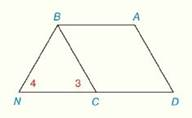Chapter 4.CR, Problem 29CRElementary Geometry For College St...

7th Edition
Alexander + 2 others
ISBN: 9781337614085

Solutions

Chapter
SectionElementary Geometry For College St...

7th Edition
Alexander + 2 others
ISBN: 9781337614085
Textbook Problem

Review Exercises Given: A B C D is a parallelogram D C - ≅ B N - ∠ 3 ≅ ∠ 4 Prove: A B C D is a rhombusTo determine

To Prove:

The provided quadrilateral ABCD is a rhombus.

Explanation

Proof:

Definition:

A rhombus is a parallelogram with two congruent adjacent sides.

Theorem on parallelogram:

The opposite sides of a parallelogram are congruent.

Description:

It is given that ABCD is a parallelogram with DC¯BN¯ and 34.

Since 34, BCN is an isosceles triangle with BN=BC

Still sussing out bartleby?

Check out a sample textbook solution.

See a sample solution

The Solution to Your Study Problems

Bartleby provides explanations to thousands of textbook problems written by our experts, many with advanced degrees!

Get Started

Prove that limx15(x+1)3=.

Single Variable Calculus

.

Mathematical Applications for the Management, Life, and Social Sciences

Define the terms population and sample, and explain the role of each in a research study.

Essentials of Statistics for The Behavioral Sciences (MindTap Course List)

In Exercises 4756, solve the given equation for the indicated variable. 27=32x1

Finite Mathematics and Applied Calculus (MindTap Course List)

Find the general indefinite integral. (u62u5u3+27)du

Single Variable Calculus: Early Transcendentals

For a given M 0, the corresponding in the definition of limx012x4= is: a) 2M4 b) 2M4 c) 12M4 d) 12M4

Study Guide for Stewart's Single Variable Calculus: Early Transcendentals, 8th